# Grade 5 Math STAAR Review Activities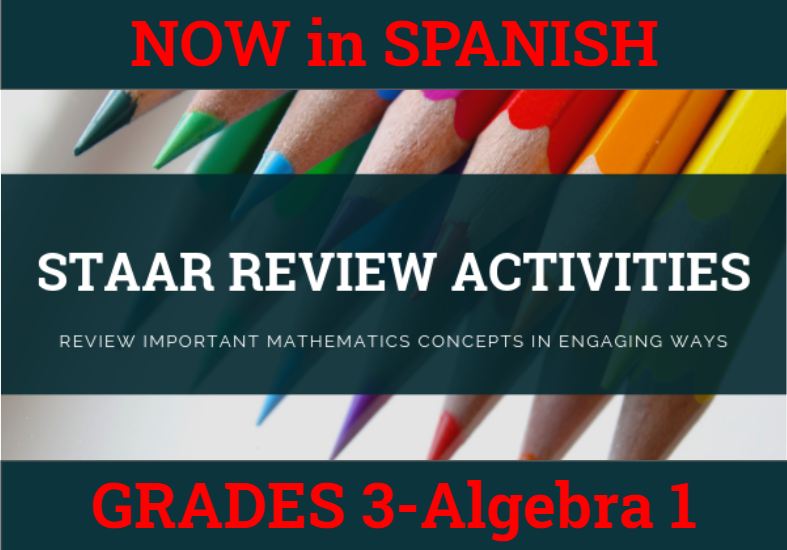How might we purposefully and effectively review important mathematics concepts for the Grade 5 Math STAAR assessment? The ESC-18 Math Team has created a variety of activities where students practice and apply important grade-level TEKS aligned topics to cement their learning.

### Please See Our Pricing and Instructions to Purchase our STAAR Activities

 5.2B Comparing & Ordering Decimals Dominoes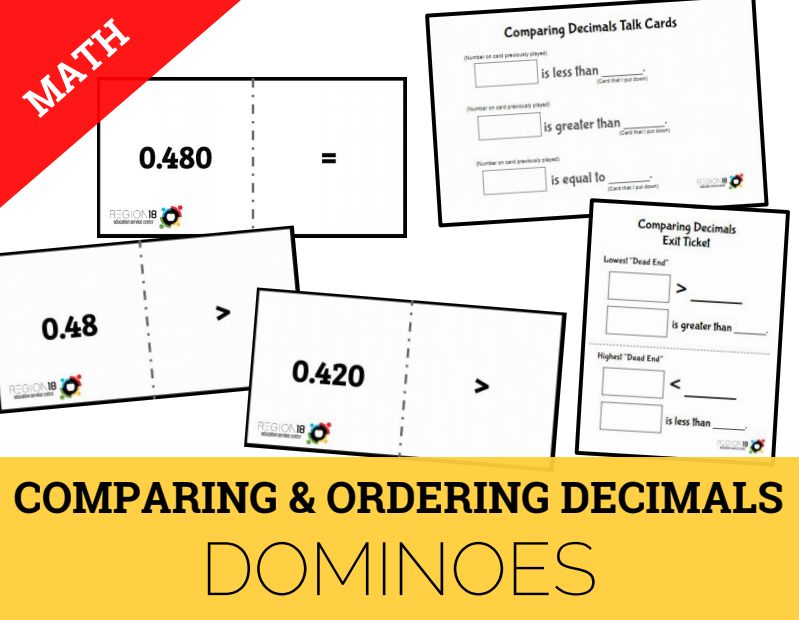Compare and order two decimals to thousandths and represent comparisons using the symbols >, <, or =. 5.2BComparing Decimals True/False Sort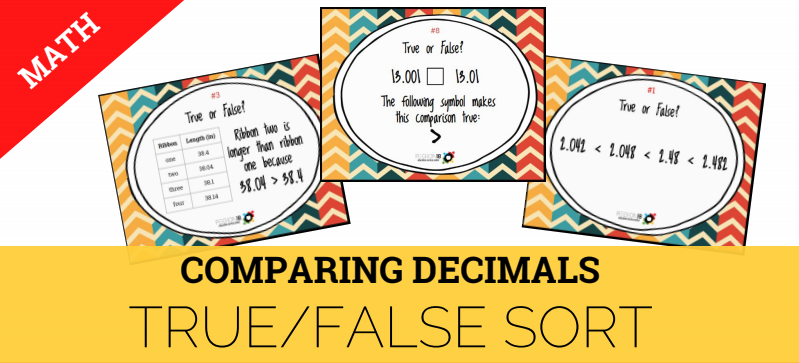Compare and order two decimals to thousandths and represent comparisons using the symbols >, <, or =. 5.3E 5.3G Multiplying and Dividing with Decimals Question Stack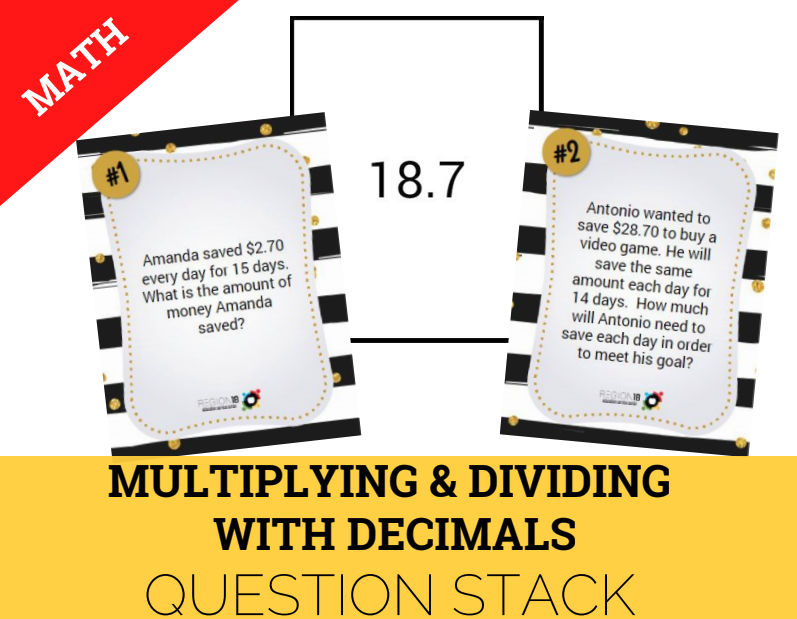Solve for products and quotients of decimals to the hundredths using strategies and algorithms. 5.3K Adding and Subtracting Positive Rational Numbers Square Puzzle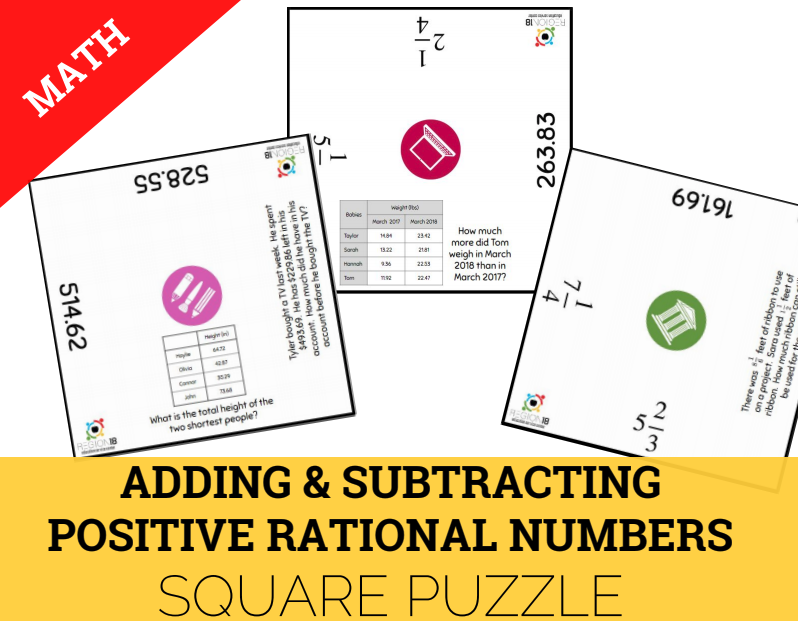Add and subtract positive rational numbers fluently. 5.3L Dividing with Fractions Task Cards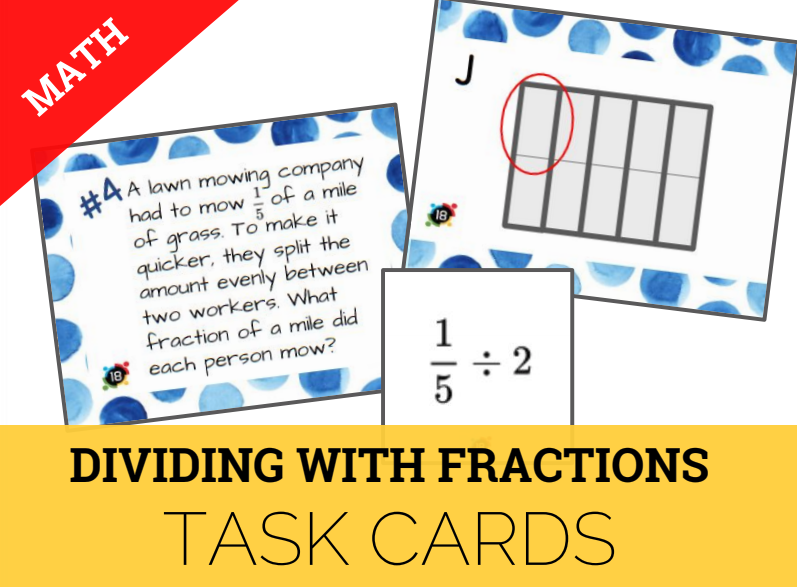Represent and solve division of whole numbers by unit fractions and unit fractions by whole numbers. 5.4B Multi-Step Problems using Operations Paper Chain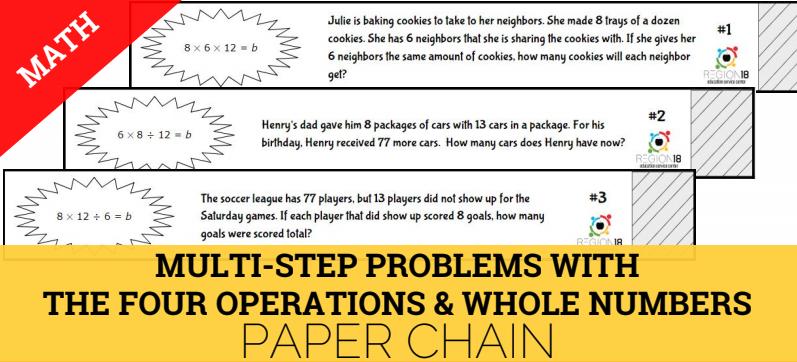Represent and solve multi-step problems involving the four operations with whole numbers using equations with a letter standing for the unknown quantity. 5.4C Representing Equations with Graphs & Tables Card Sort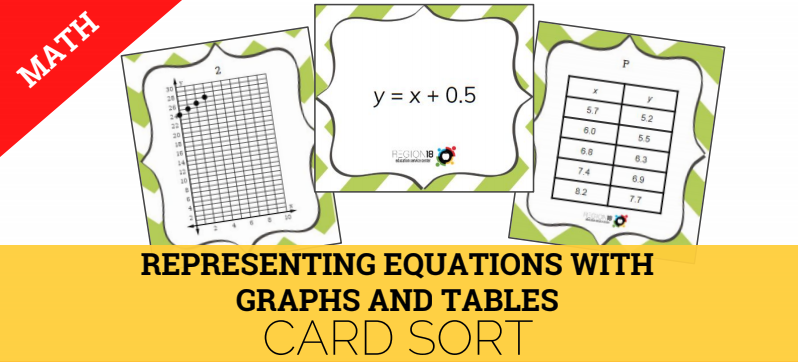Generate and graph a numerical pattern when given a rule in the form y = ax or y = x + a. 5.4F Simplifying Numerical Expressions Quick Flips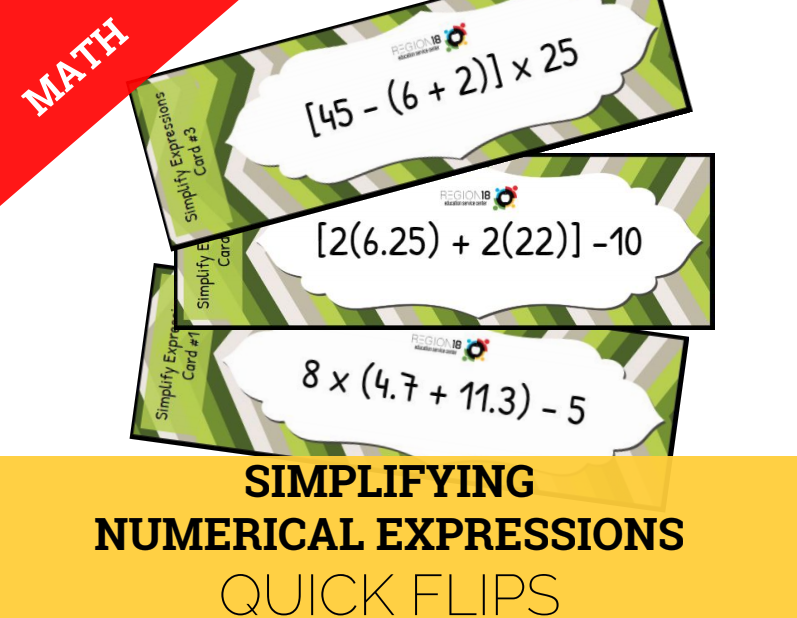Simplify numerical expressions that do not involve exponents, including up to two levels of grouping. 5.4H Perimeter, Area, and Volume Sum It Up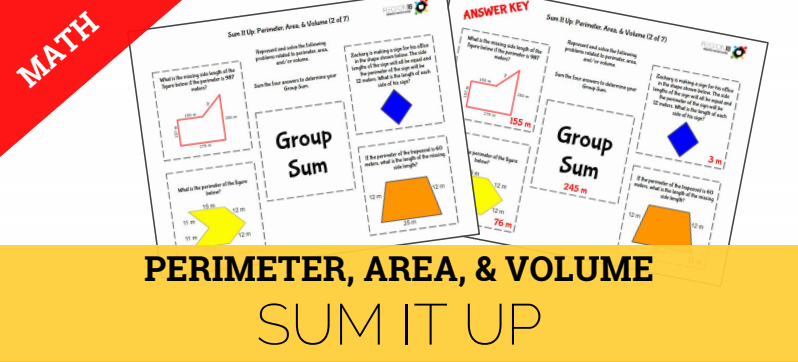Represent and solve problems related to perimeter and/or area and related to volume. 5.5A Classifying Two-Dimensional Figures Shape Challenge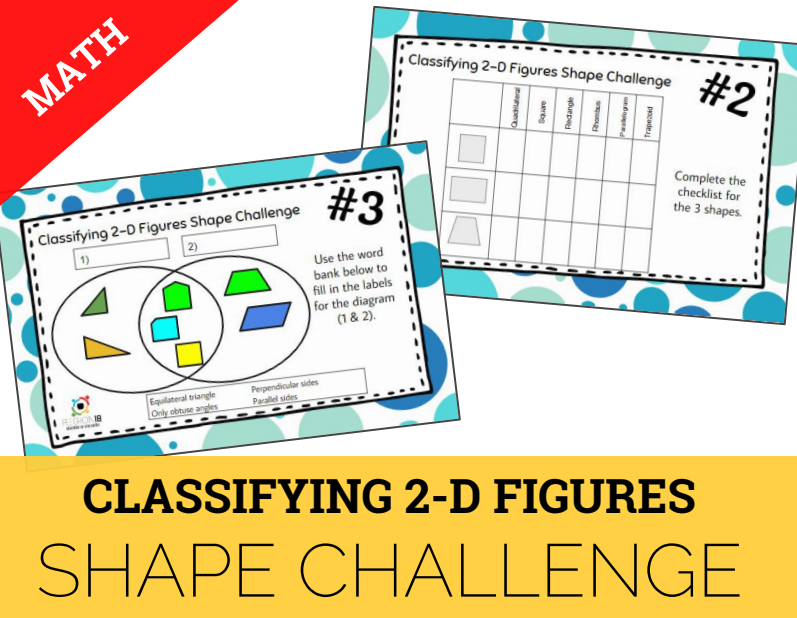Classify two-dimensional figures in a hierarchy of sets and subsets using graphic organizers based on their attributes and properties. 5.8C Graphs, Tables, & Ordered Pairs Match & Snip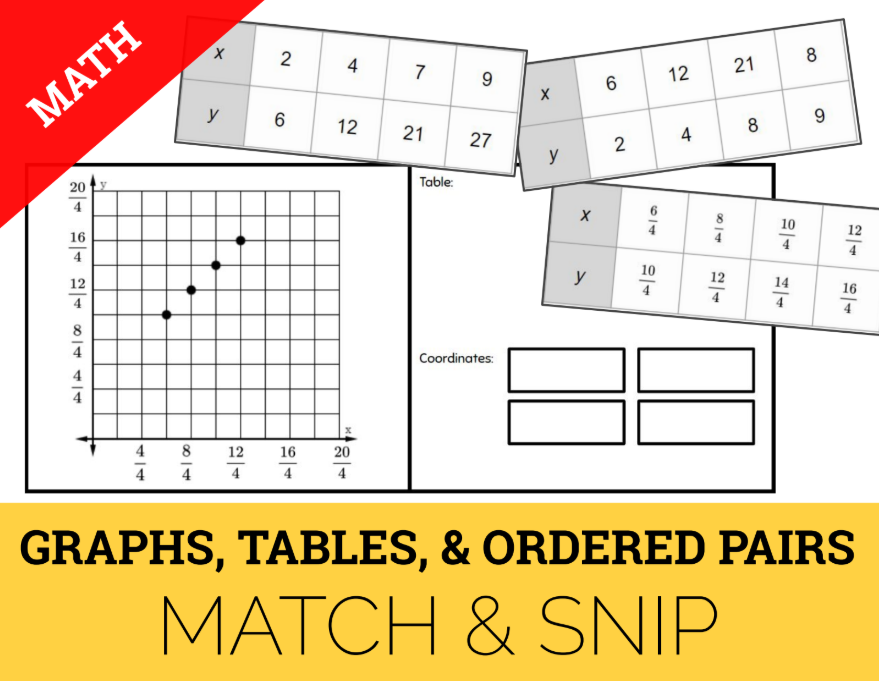Graph in the first quadrant of the coordinate plane ordered pairs of numbers arising from mathematical and real-world problems, including those generated by number patterns or found in an input-output table. 5.9C Problem Solving with Data Representations Scavenger Hunt Solve one- and two-step problems using data from a frequency table, dot plot, bar graph, stem-and-leaf plot, or scatterplot.